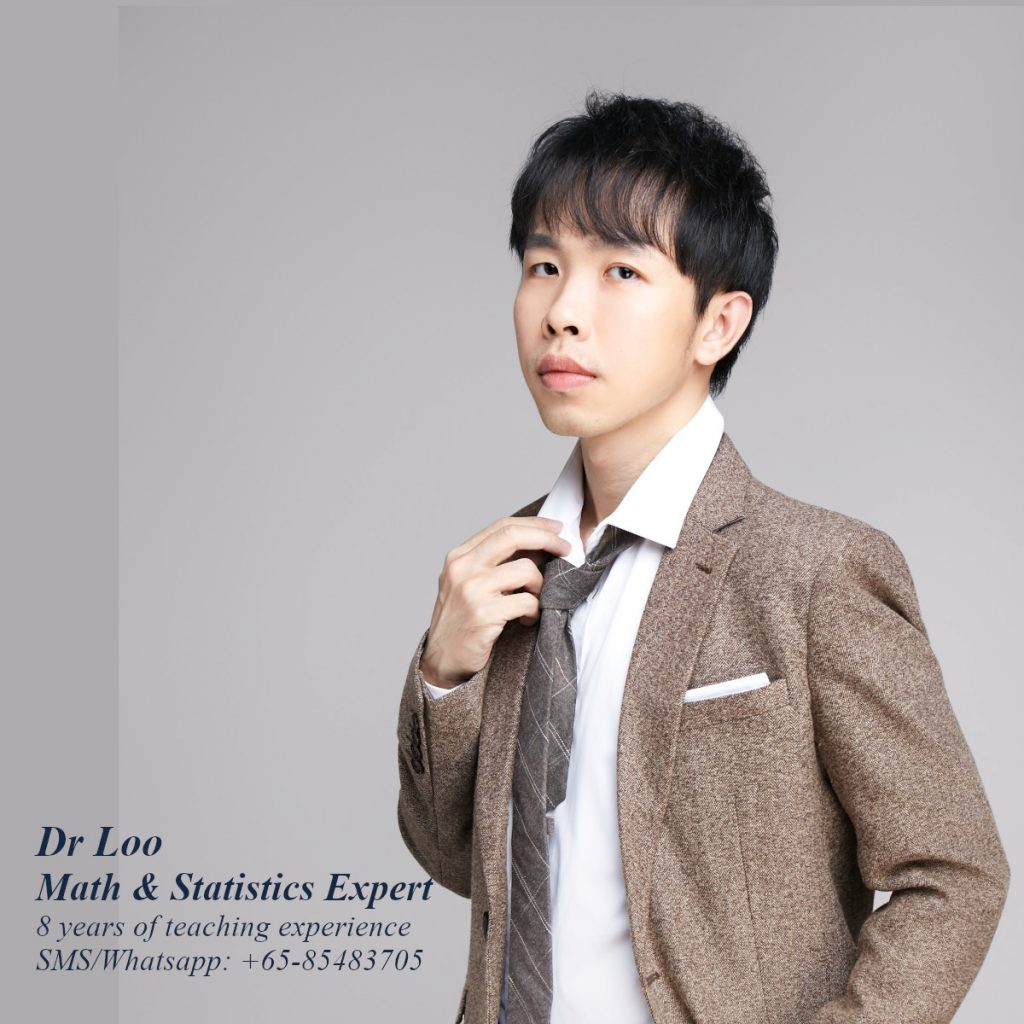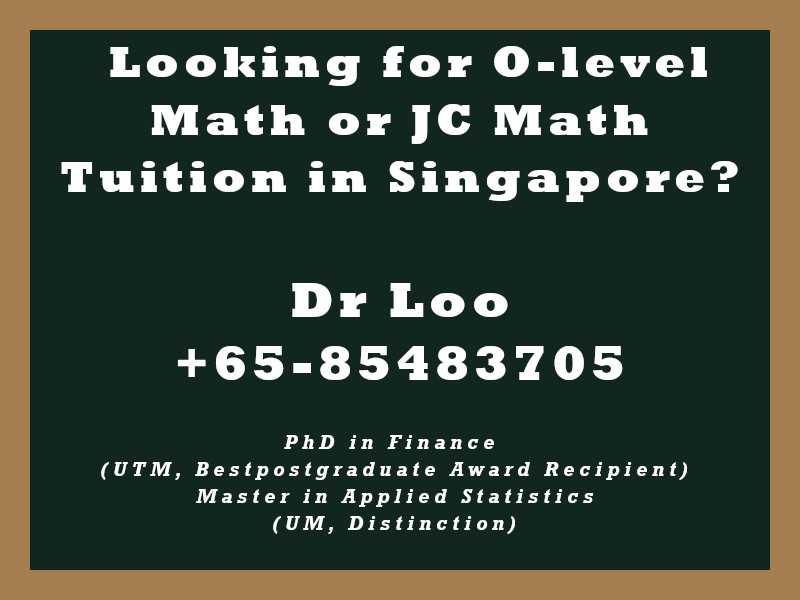+65-85483705
Select Page

# Simple Linear Regression Analysis

## 1. Simple Regression

This can be done by regressing dependent variable to the independent variables. This concept is likely to be introduce during the JC H2 mathematics class.

## 2. The general notation of a simple regression model

y = B0 + B1x + u

Where,

• y is the dependent variable
• x is the independent variable
• B0 is the intercept
• B1 is the slope

### 3. Formula of calculating regression slope in simple regression

A regression slope can be calculated using ordinary least square method. This method is to obtain the regression slope that can minimize the sum of square between the predicted dependent variable and the true dependent variable.

Regression slope, beta = Covariance(x,y)/Variance(x)

Question

Determine the regression slope for the following datasets.

Question 1

 y1 x1 0 3 5 1 2 1 5 3 0 3 2 2 3 4 4 0 0 1 1 4 4 0 1 1 4 5 3 1

Question 2

 y2 x2 4 3 5 5 4 5 2 3 2 5 5 2 0 0 5 2 2 1 3 1 4 3 4 4 2 0 1 2

Question 3

 y3 x3 5 4 1 3 0 4 4 1 4 5 4 1 3 0 4 1 1 4 1 3 2 4 5 1 3 2 4 4

Question 4

 y4 x4 5 3 5 0 3 4 2 3 0 2 1 1 3 4 1 0 0 5 0 2 5 3 1 1 5 3 0 5

Question 5

 y5 x5 1 0 1 4 2 0 1 4 0 1 4 5 4 0 4 0 3 4 4 1 0 0 2 0 0 3 0 0

1. -0.134
2. 0.442
3. -0.342
4. -0.077
5. 0.064

Notes: There are other useful information can be determined when performing simple regression analysis such as the R-squared.

## About the Math Tutor - Dr Loo

I am a PhD holder with 9 years of teaching experience at secondary school and university. My expertizes are mathematics, statistics, econometrics, finance and machine learning.

Currently I do provide consultation and private tuition in Singapore. For those who are looking for math tutor in Singapore or statistics tutor in Singapore, please feel free to contact me at +65-85483705 (SMS/Whatsapp/Telegram).

Some of the math tuition in Singapore I provide includes O-level math tuition and also JC Math Tuition (H1 Math Tuition & H2 Math Tuition). On the other hand, my statistics tuition in Singapore mostly focus on pre-university level until postgraduate level. For those who prefer online tutoring service, the online O-level math tuition and online JC math tuition are also available.## For those who are looking for math tution in Singapore

Need help with this topic? I do provide mathematics home tuition in Singapore for O-level math and also JC H2 math. In addition, online math tutoring is available as well. Feel free to contact me if you would like to know further.